﻿ 蜂巢砌块挡土墙结构安全性分析

# 蜂巢砌块挡土墙结构安全性分析Safety Analysis of Retaining Wall Structure in Honeycomb Block

Abstract: Retaining wall in Honeycomb block is an ecological retaining wall. It combines the advantages of gravity retaining wall and cantilever retaining wall from the structural point of view. It has the advantages of quick and convenient construction, controllable quality and beautiful appearance, and excellent ecological effects and other advantages. In this paper, the finite element method is used to analyze the nonlinear contact stress distribution between the soil and the structure of the retaining wall in honeycomb block to confirm the structural stability of the ecological retaining wall. The results show that the soil arching phenomenon occurs in the soil pressure behind the retaining wall of the honeycomb block. The soil pressure near the height of 1/3 retaining wall is higher than the active earth pressure, but the combined force of the soil pressure and the action point and the Coulomb active earth pressure are basically the same. The stress distribution of the wall is similar to that of the cantilever beam, and stress concentration occurs at the joint of the block.

1. 引言

2. 工程概况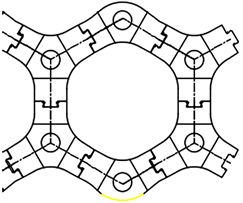Figure 1. The cross section of honeycomb block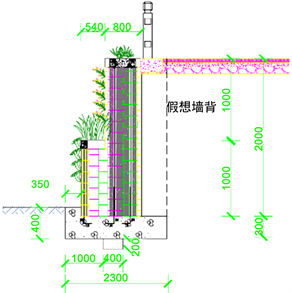Figure 2. The cross section of retaining wall

3. D-P系列屈服准则

1900年摩尔教授(O. Mohr)提出了著名的Mohr-Coulomb强度理论(简称M-C强度理论)，能够较好的描述岩土材料的强度特性，在岩土工程中得到了广泛的应用。但是，M-C准则在三维空间的屈服面为π平面上的不等角六边形，给数值计算带来了很大的困难。

Drucker-Prager屈服准则(简称D-P准则)通过改变π平面中Mises圆的大小来逼近M-C准则，便于进行数值计算 。

$F=\frac{1}{3}{I}_{1}\mathrm{sin}\phi +\left(\mathrm{cos}{\theta }_{\sigma }-\frac{1}{3}\mathrm{sin}{\theta }_{\sigma }\mathrm{sin}\phi \right)\sqrt{{J}_{2}}-c\mathrm{cos}\phi =0$ (1)

$\alpha {I}_{1}+\sqrt{{J}_{2}}=k$ (2)

$\alpha =\frac{\mathrm{sin}\phi }{\sqrt{3}\left(\sqrt{3}\mathrm{cos}{\theta }_{\sigma }-\mathrm{sin}{\theta }_{\sigma }\mathrm{sin}\phi \right)}$ (3)

$k=\frac{\sqrt{3}c\mathrm{sin}\phi }{\sqrt{3}\mathrm{cos}{\theta }_{\sigma }-\mathrm{sin}{\theta }_{\sigma }\mathrm{sin}\phi }$ (4)

${\theta }_{\sigma }=a\mathrm{tan}\left(\frac{2{\sigma }_{2}-{\sigma }_{1}-{\sigma }_{3}}{\sqrt{3}\left({\sigma }_{1}-{\sigma }_{3}\right)}\right)$ (5)

$c$$\phi$ 为材料的粘聚力和内摩擦角， ${\theta }_{\sigma }$ 则反映了材料的受力状态。由于逼近的准则不同，D-P准则有很多不同的修正准则：外角点外接圆准则(DP1)、内角点外接圆准则(DP2)、内切圆准则(DP3)、等面积圆准则(DP4)、M-C匹配DP圆准则(DP5)。D-P准则在对应的应力洛德角在与材料受力状态一致或者相近时能够得到理想的结果。在单向压缩及常规三轴压缩状态下( ${\theta }_{\sigma }=\text{π}/6$ )，DP1和M-C准则是相匹配的；单向拉伸及常规三轴拉伸状态下( ${\theta }_{\sigma }=-\pi /6$ )，DP2和M-C相匹配；平面应变条件的关联流动法则下

( ${\theta }_{\sigma }=a\mathrm{tan}\left(-\frac{\mathrm{sin}\phi }{\sqrt{3}}\right)$ )，DP3和M-C相匹配；非关联流动法则下( ${\theta }_{\sigma }=0$ )，DP5与M-C相匹配 。

4. 计算模型设计

4.1. 模型建立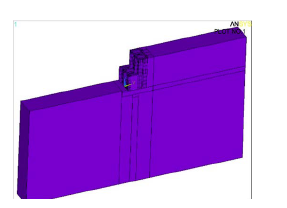Figure 3. Calculation model of retaining wall in honeycomb block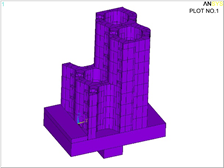Figure 4. The real retaining wall in honeycomb block

4.2. 模型参数

4.3. 模型单元选择

4.4. 模型边界条件

5. 计算结果分析

5.1. 挡墙墙后土压力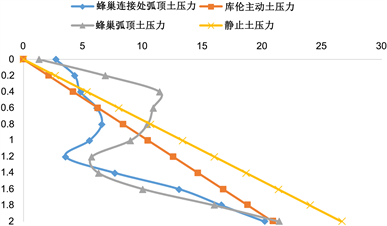Figure 5. Horizontal earth pressureFigure 6. The earth pressure contours in the horizontal direction

5.2. 挡墙位移分析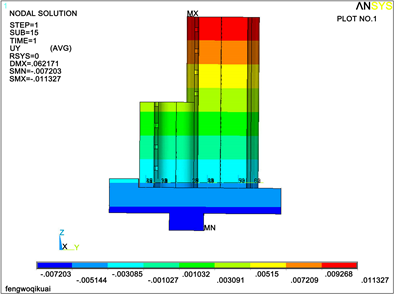Figure 7. The displace of retaining wall in the horizontal direction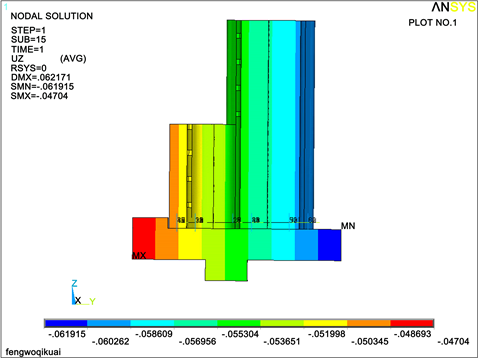Figure 8. The displace of retaining wall in the vertical direction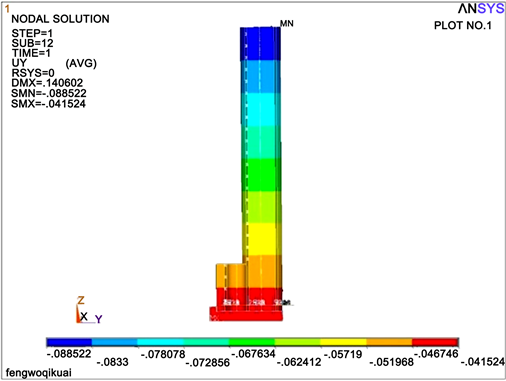Figure 9. Horizontal displacement of the retaining wall with the heightFigure 10. Verticaldisplacement of the retaining wall with the height

5.3. 挡墙应力分析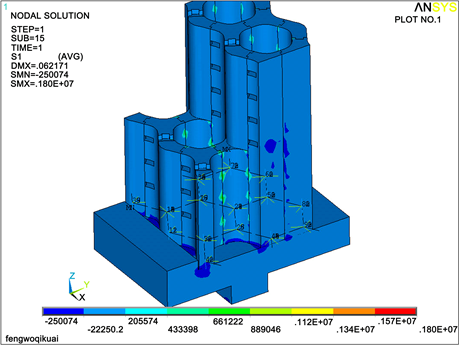Figure 11. The distribution of first principal stress on the front of retaining wall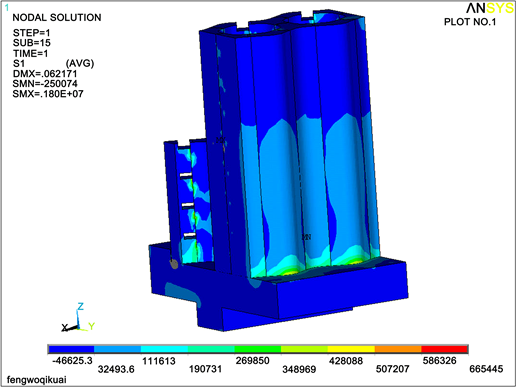Figure 12. The distribution of first principal stress on the back of retaining wall

6. 结论

1) 蜂巢砌块挡墙的墙背水平土压力由于土拱效应的影响呈倒S形分布，土压力合力要小于等于库伦主动土压力合力，且作用点位于距离底部0.34 H的地方。所以实际工程中仍然可以按照库伦土压力理论进行设计计算，出于安全考虑可以在库伦主动土压力的基础上乘以1.2的安全系数。

2) 挡土墙整体重心靠近墙踵，虽然可以提供较大的抗倾覆力矩，但是也会带来基础应力偏心距较大而造成不均匀沉降的危险，设计截面时要注意墙体重心的位置，可以适当提高墙面筒的高度或者延长底板进行平衡。

3) 挡墙受力形式类似于悬臂梁受弯，主要危险点在墙背受拉和砌块拼接部分的应力集中。在实际工程中，需要注意在砌块中穿结的钢筋和底板的锚固以及砌块拼接部分进行适当的补强。

 SL379-2007. 水工挡土墙设计规范[S].

 吕凤梧. 深基坑施工过程多支撑挡土墙动态可靠度计算[J]. 工业建筑, 2003, 33(7): 1-5.

 邢肖鹏. 挡土墙土压力有限元模拟分析[J]. 山西水利科技, 2002, 146(4): 1-3.

 苏利亚. 二级悬臂式挡土墙的力学分析[J]. 黑龙江交通科技, 2017, 40(5): 10-11.

 施小平. 悬臂式挡土墙抗滑稳定性分析[J]. 南水北调与水利科技, 2015, 13(1): 108-112.

 陈忠达, 汪东升. 悬锚式挡土墙有限元分析[J]. 公路交通科技, 2005, 22(1): 38-41.

 邓楚建, 何国杰. 基于M-C准则的D-P系列准则在岩土工程中的应用研究[J]. 岩土工程学报, 2006, 28(6): 736-739.

 王先军, 陈明祥. Drucker-Prager系列屈服准则在稳定分析中的应用研究[J]. 岩土力学, 2009, 30(12): 3734-3738.

 王国田, 罗雪贵. 滑坡-抗滑桩土拱效应三维数值模拟研究[J]. 路基工程, 2018, 199(4): 90-94.

 吴昌将, 张子新. 边坡工程中抗滑桩群桩土拱效应的数值分析[J]. 地下空间与工程学报, 2007, 3(7): 1305-1333.

 王诚, 石少卿. 基于法向应力突变的组合式钢管抗滑桩土拱效应模型试验研究[J]. 科学技术与工程, 2016, 16(10): 1671-1815.

Top